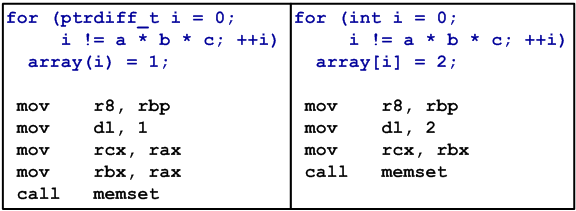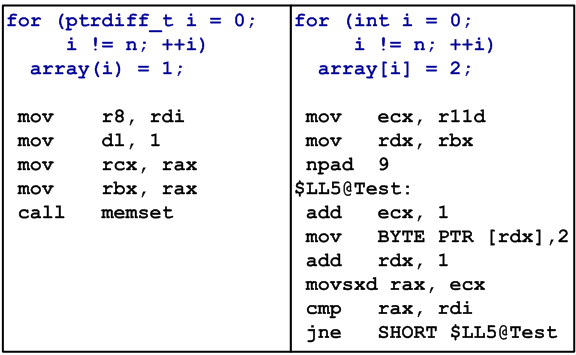﻿ Optimization in the world of 64-bit errors# Optimization in the world of 64-bit errors

In the previous blog-post I promised to tell you why it is difficult to demonstrate 64-bit errors by simple examples. We spoke about operator[] and I told that in simple cases even incorrect code might work. Here is such an example:

``````class MyArray
{
public:
char *m_p;
size_t m_n;
MyArray(const size_t n)
{
m_n = n;
m_p = new char[n];
}
~MyArray() { delete [] m_p; }
char &operator[](int index)
{ return m_p[index]; }
char &operator()(ptrdiff_t index)
{ return m_p[index]; }
ptrdiff_t CalcSum()
{
ptrdiff_t sum = 0;
for (size_t i = 0; i != m_n; ++i)
sum += m_p[i];
return sum;
}
};
void Test()
{
ptrdiff_t a = 2560;
ptrdiff_t b = 1024;
ptrdiff_t c = 1024;
MyArray array(a * b * c);
for (ptrdiff_t i = 0; i != a * b * c; ++i)
array(i) = 1;
ptrdiff_t sum1 = array.CalcSum();
for (int i = 0; i != a * b * c; ++i)
array[i] = 2;
ptrdiff_t sum2 = array.CalcSum();
if (sum1 != sum2 / 2)
MessageBox(NULL, _T("Normal error"),
_T("Test"), MB_OK);
else
MessageBox(NULL, _T("Fantastic"),
_T("Test"), MB_OK);
}``````

Briefly, this code does the following:

• Creates an array of 2.5 Gbytes (more than INT_MAX items).
• Fills the array with ones by using the correct operator() with ptrdiff_t parameter.
• Calculates the sum of all the items and writes it into the variable sum1.
• Fills the array with twos by using the incorrect operator[] with int parameter. Theoretically, int does not allow us to address the items whose numbers are more than INT_MAX. There is one more error in the loop "for (int i = 0; i != a * b * c; ++i)". Here, we also use int as the index. This double error is made for the compiler not to generate warnings about a 64-bit value converting to a 32-bit one. Actually, an overflow and addressing an item with a negative number must take place what will result in a crash. By the way, this is what happens in the debug-version.
• Calculates the sum of all the items and writes it into the variable sum2.
• If (sum1 == sum2 / 2), it means that the impossible became true and you see the message "Fantastic".

Despite the two errors in this code, it successfully works in the 64-bit release-version and prints the message "Fantastic"!

Now let us make out why. The point is that the compiler guessed our wish to fill the array with the values 1 and 2. And in the both cases it optimized our code by calling memset function:The first conclusion is: the compiler is a clever guy in the questions of optimization. The second conclusion - stay watchful.

This error might be easily detected in the debug-version where there is no optimization and the code writing twos into the array leads to a crash. What is dangerous, this code behaves incorrectly only when dealing with large arrays. Most likely, processing of more than two milliards of items will not be present in the unit-tests run for the debug-version. And the release-version might keep this error a secret for a long time. The error can occur quite unexpectedly at a slightest change of the code. Look what can happen if we introduce one more variable, n:

``````void Test()
{
ptrdiff_t a = 2560;
ptrdiff_t b = 1024;
ptrdiff_t c = 1024;
ptrdiff_t n = a * b * c;
MyArray array(n);
for (ptrdiff_t i = 0; i != n; ++i)
array(i) = 1;
ptrdiff_t sum1 = array.CalcSum();
for (int i = 0; i != n; ++i)
array[i] = 2;
ptrdiff_t sum2 = array.CalcSum();
...
}``````

The release-version crashed this time. Look at the assembler code.The compiler again built the code with a memset call for the correct operator(). This part still works well as before. But in the code where operator[] is used, an overflow occurs because "i != n" condition does not hold. It is not quite the code I wished to create but it is difficult to implement what I wanted in a small code while a large code is difficult to examine. Anyways, the fact remains. The code now crashes as it should be.

Why have I devoted so much time to this topic? Perhaps I am tormented with the problem that I cannot demonstrate 64-bit errors by simple examples. I write something simple for the purpose of demonstration and what a pity it is when one tries it and it works well in the release-version. And therefore it seems that there is no error. But there are errors and they are very insidious and difficult to detect. So, I will repeat once again. You might easily miss such errors during debugging and while running unit-tests for the debug-version. Hardly has anyone so much patience to debug a program or wait for the tests to complete when they process gigabytes. The release-version might pass a large serious testing. But if there is a slight change in the code or a new version of the compiler is used, the next build will fail to work at a large data amount.

To learn about diagnosis of this error, see the previous post where the new warning V302 is described.##### Use PVS-Studio to search for bugs in C, C++, C# and Java

We offer you to check your project code with PVS-Studio. Just one bug found in the project will show you the benefits of the static code analysis methodology better than a dozen of the articles.

409
14 072

### Do you make errors in the code?